# Algebra II: Graphing and Factoring Quadratic Equations Review Chapter Exam

Exam Instructions:

Choose your answers to the questions and click 'Next' to see the next set of questions. You can skip questions if you would like and come back to them later with the yellow "Go To First Skipped Question" button. When you have completed the practice exam, a green submit button will appear. Click it to see your results. Good luck!

### Page 1

#### Question 4 4. When completing the square for the quadratic equation shown below, how is c calculated?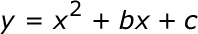#### Question 5 5. What kind of graph is shown?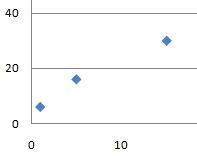### Page 3

#### Question 11 11. If the equation below told you a ball was 'h' feet in the air after 't' seconds, what would be the first step to finding out when it hit the ground?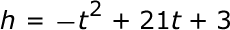### Page 4

#### Question 16 16. Factor and solve.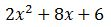#### Question 17 17. Factor and solve.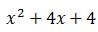#### Question 18 18. Expressing the equation below in the vertex form will give a value of 'h' = _____.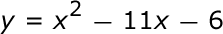#### Question 20 20. Find the solution to the equation below.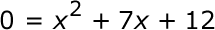### Page 5

#### Question 24 24. Rewrite the following equation in vertex form.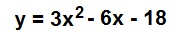### Page 6

#### Question 29 29. Find the value for c that must be added to both sides, and rewrite the equation in the vertex form.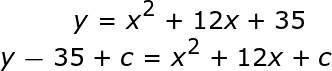#### Algebra II: Graphing and Factoring Quadratic Equations Review Chapter Exam Instructions

Choose your answers to the questions and click 'Next' to see the next set of questions. You can skip questions if you would like and come back to them later with the yellow "Go To First Skipped Question" button. When you have completed the practice exam, a green submit button will appear. Click it to see your results. Good luck!

Support